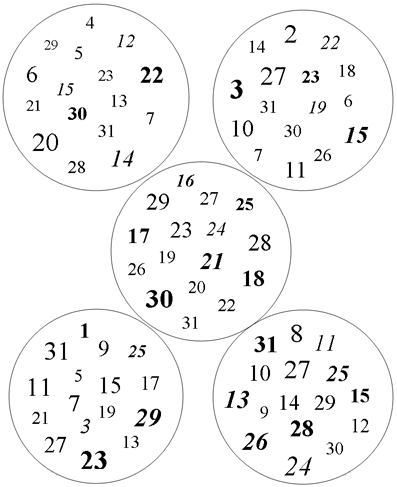beginning of content:

Contributed by Jesse Lubinski
Irvington High School
Irvington, New York

Adapted from material by Jeff Chertok.

This is a great introductory lesson to binary numbers. Distribute a copy of the circles image below to each student in the class. You can begin by telling the students that in addition to being a teacher you are also a mind reader. To prove it, you will perform a demonstration of your "magic."

## How to Perform the Trick:

1. Ask a student to pick a number between 1 and 31, inclusive. Make sure the student does not say out loud the number they have chosen.
2. The key to performing this trick is to memorize the top number of each circle (4 in the upper left, 2 in the upper right, 16 in the center, 1 in the lower left, 8 in the lower right).
3. Beginning with the first circle (the one in the upper left), ask the student if the number they are thinking of is in the circle. You are going to begin keeping a running total. If the student says that the number they are thinking of is in the circle you are asking about, you will add the top number of that circle to the total.
4. Repeat step 3 for all five of the circles.
5. After you have checked all five circles, the total you have accumulated will be the number the student has been thinking of. Casually tell the student what their number is and watch the students react in awe. Well, not awe exactly—but they like the trick.

## How the Trick Works:

The trick is based on binary numbers. Binary numbers are numbers in which each digit is represented by only two digits, 0 or 1. In computing, they are used by computers for memory, storage, processing, communications, or any other place where two different states are used. The 0 and 1 values are sometimes called "low" and "high," respectively.

The number system we normally use is written in Base 10, meaning that each digit is a power of ten. For instance, the number 149 has a 1 in the hundreds (102) place, a 4 in the tens (101) place, and a 9 in the ones (100) place. It can be viewed as:

102 101 100
1 4 9

Another way of thinking about Base 10 is that we have ten choices for each digit. Since we know that binary numbers have only two choices, 0 or 1, we can call them Base 2 numbers. Therefore, each place value would be a power of two. So the number 149 would be:

27 26 25 24 23 22 21 20
1 0 0 1 0 1 0 1

Look at the columns with the 1s. They are 27 (128), 24 (16), 22 (4), and 20 (1). When we add these together (128 + 16 + 4 + 1) we get 149.

If we take a look at the numbers from the trick (1 through 31, inclusive) in binary form, we can see the following:

Decimal 24 23 22 21 20 Binary
1 0 0 0 0 1 00001
2 0 0 0 1 0 00010
3 0 0 0 1 1 00011
4 0 0 1 0 0 00100
5 0 0 1 0 1 00101
6 0 0 1 1 0 00110
7 0 0 1 1 1 00111
8 0 1 0 0 0 01000
9 0 1 0 0 1 01001
10 0 1 0 1 0 01010
11 0 1 0 1 1 01011
12 0 1 1 0 0 01100
13 0 1 1 0 1 01101
14 0 1 1 1 0 01110
15 0 1 1 1 1 01111
16 1 0 0 0 0 10000
17 1 0 0 0 1 10001
18 1 0 0 1 0 10010
19 1 0 0 1 1 10011
20 1 0 1 0 0 10100
21 1 0 1 0 1 10101
22 1 0 1 1 0 10110
23 1 0 1 1 1 10111
24 1 1 0 0 0 11000
25 1 1 0 0 1 11001
26 1 1 0 1 0 11010
27 1 1 0 1 1 11011
28 1 1 1 0 0 11100
29 1 1 1 0 1 11101
30 1 1 1 1 0 11110
31 1 1 1 1 1 11111

Notice that each power of two has 16 numbers that have a 1 for that particular bit. The way the trick is formed is by treating each bit (or power of two) as one of the circles in the trick. This means that each circle will have 16 numbers in them. If a number has a 1 for that bit, it is added to the circle. So according to our chart, the number 31 will appear in all five circles. The number 20 will appear in two circles, 24 (16) and 22 (4), which added together equals 20. The key is that the top number of each circle is the actual value of that place value. If the student correctly identifies all of the circles in which a number appears, adding the top numbers (place values) will help you correctly identify the number.

Note that the different fonts and sizes of the numbers in the circles do not actually matter. They are there to simply add some confusion to those who are trying to figure the trick out since they often mistake the sizes of the numbers to have meaning.

To take a look at an algorithm, along with pseudocode, which helps explain how to convert decimal to binary and vice versa, visit the Binary System Web site.